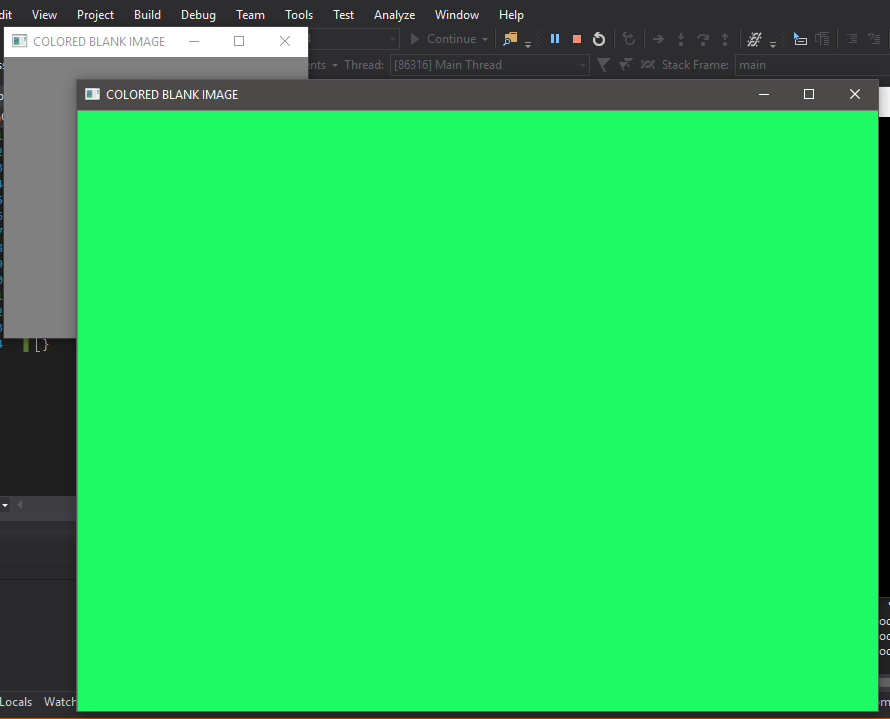Related Articles

# OpenCv | Coloured Blank Image

• Last Updated : 26 Aug, 2019

This article aims to learn how to load a blank coloured image using OpenCv. Using OpenCV, we can generate a blank image with any colour one wishes to.
So, let us dig deep into it and understand the concept with the complete explanation.

### Code : C++ code for creating blank colored image

 `// c++ code explaining how to``// open a blank colored image``// in OpenCV`` ` `// loading library files``#include ``#include ``#include `` ` `using` `namespace` `cv;``using` `namespace` `std;`` ` `int` `main(``int` `argc, ``char``** argv)``{``    ``// Creating the image``    ``Mat img(600, 800, CV_8UC3, Scalar(100, 250, 30));`` ` `    ``// Naming the window``    ``String geeks_window = ``"COLORED BLANK IMAGE"``;`` ` `    ``// crearting window for image display``    ``namedWindow(geeks_window);`` ` `    ``// image shown inside the window``    ``imshow(geeks_window, img);`` ` `    ``// wait for any key press``    ``waitKey(0);`` ` `    ``// destroying the created window``    ``destroyWindow(geeks_window);``    ``return` `0;``}`

Output :### Explanation :

 `// Creating the image``Mat img(600, 800, CV_8UC3, Scalar(100, 250, 30));`

No. of rows in the image i.e. height
rows = 100

No. of columns in the image i.e. width
columns = 250

Data Type i.e. depth. There are a lot of arguments for a type
type = CV_8UC3 (we have randomly chosen one of the possible arguments)

Value of Blue, Green and Red channels
scalar = (100, 250, 30)

It is constructor available in Mat Class and it creates image with selected rows and columns as arguments. An image created this way has around 24 bits for each pixel as it allocates 8 bit integer value representing RGB – red, green and blue planes respectively. The value of these three integers can vary from 0(black) to 255(white). Here in the code above, each pixel of the newly created image is initialized with a constructor to 3 unsigned integers i.e. scalar values – 100, 250, 30. This means it has assigned blue, green and red channel with 100, 250, 30 respectively. As you can see the image is kind of greenish as the value for green channel is a lot higher. The image is a combination of these three colour values.

My Personal Notes arrow_drop_up